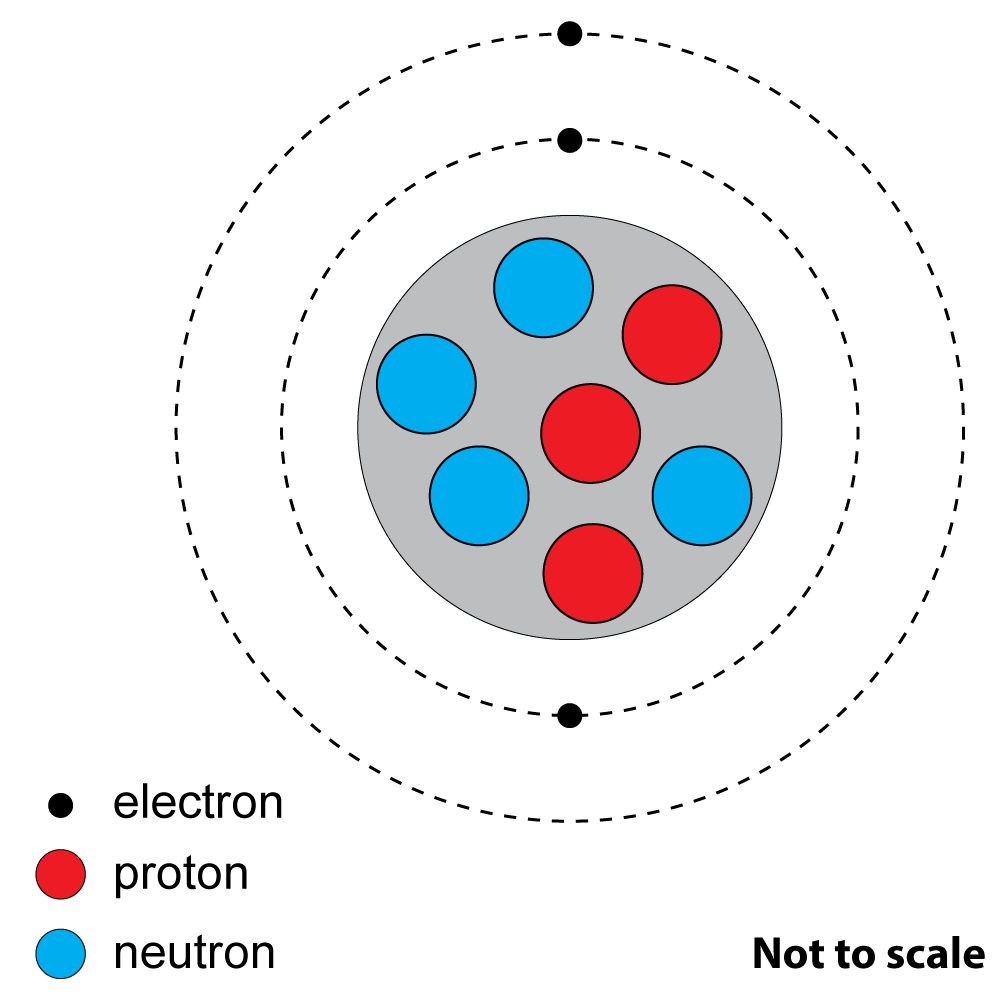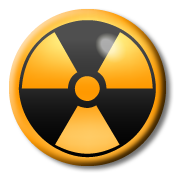1. An atom of 14 C contains electrons, protons and neutrons. 6

How many?

 number of electrons number of protons number of neutrons A. 14 6 14 B. 14 6 8 C. 6 8 6 D. 6 6 8

2+3: This is a diagram of an atom of a common element.2. What is the atomic number?

A). 3
B). 4
C). 7
D). 10

3. What is the nucleon (mass) number?

A). 3
B). 4
C). 7
D). 10

4. Two isotopes of the same element have ...

A). the same number of protons but different numbers of neutrons.
B). the same number of protons but different numbers of electrons.
C). the same number of neutrons but different numbers of protons.
D). the same number of electrons but different numbers of protons.

5. An atom that has been ionised has ...

A). Lost or gained protons
B). Lost or gained electrons
C). Lost or gained a nucleon
D). Lost or gained a neutron

6. A radioactive isotope is a substance that ...

A). will eventually gain electrons through bonding.
B). will become stable by cooling down.
C). is unstable and will decay by losing particles.

Which type(s) of radiation match the following descriptions?7. The most ionising.
8. A fast moving electron.
9. Can pass through paper.
11. Positively charged.
12. The greatest mass.
13. The same as a helium nucleus.
14. Deflected by an electric field.
15. The half-life of a substance is ...
• A). the amount of substance left after it has decayed.
• B). the time it takes for half of the isotope to decay.
• C). the time it takes for half the mass to have radiated away.
• D). the mass of half of the isotope after decaying.
16. Which of these is NOT a source of backgound radiation?
• A). Chemical reactions
• B). Cosmic rays
• C). Nuclear power stations
• D). Radon gas from the ground